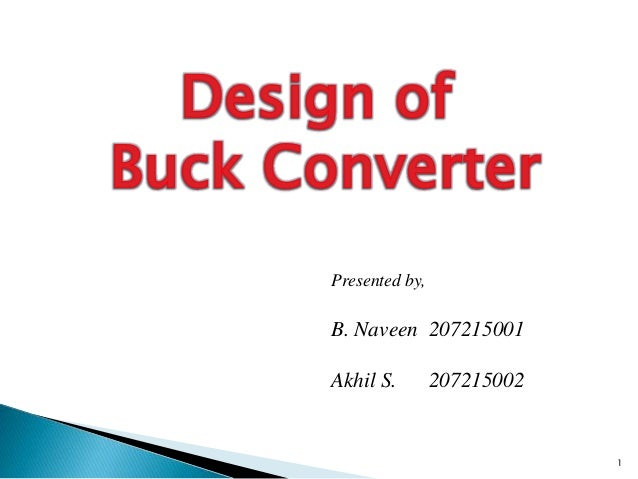Successfully reported this slideshow.
We use your LinkedIn profile and activity data to personalize ads and to show you more relevant ads. You can change your ad preferences anytime.Upcoming SlideShare
Loading in …5
×

# Design of Buck Converter

A detailed step-by-step procedure for the design of a buck converter. Different active and passive components are selected as per the requirement specified in the design problem.

• Full Name
Comment goes here.

Are you sure you want to Yes No
Your message goes here• Login to see the comments

### Design of Buck Converter

1. 1. Presented by, B. Naveen 207215001 Akhil S. 207215002 1
2. 2. Design a buck converter satisfying the following requirements:  Input Voltage, Vin=12V  Output Voltage, Vout=5V  Output Current, Io=2A  Switching frequency, fsw=400kHz  Ripple current, ΔIL=30% of IL (max.)  Ripple voltage, ΔVo=50mV (max.) 2Design of Buck converter Fig.1: Buck converter circuit arrangement
3. 3. Load resistance,RL = VO / ILOAD = 5/ 2 = 2.5X Ripplecurrent,IRIPPLE = 3 IL = 0.3 # ILOAD = 0.3 # 2 = 0.6A Output voltage, VO = DVIN ` Duty rati o, D = VIN VO = 12 5 = 0.4166 T = 1/ fsw = 400 * 10 3 1 = 2.5n sec TON = DT = 0.4166 * 2.5n = 1.04n sec TOFF = (1 - D) T = (1 - 0.4166) 2.5n = 1.45n sec 3Design of Buck converter
4. 4. 3 IL = fL D(1 - D) VS & L = f 3 IL D(1 - D) VS = 400 # 10 3 # 0.6 0.4166(1 - 0.4166) 12 = 12.152nH Also Vr ipple = 3 Vo= 8f 2 LC D(1- D)VS & C = 8f 2 L 3 Vo D(1 - D) VS = 8 # (400 # 10 3 ) 2 # 12.15 # 10 - 6 # 50 # 10 - 3 0.4166(1 - 0.4166) 12 = 3.75nF 4Design of Buck converter I max = IO + (3 IL / 2) = 2 + (0.6/ 2) = 2.3A I min = IO - (3 IL / 2) = 2 - (0.6/ 2) = 1.7A
5. 5. Critical Inductance (LC ) = 2f (1 - D) R = 2 # 400 # 10 3 (1 - 0.4166) 2.5 = 1.823nH Critical Capaci tance(CC ) = 16 # f 2 # Lc (1 - D) = 16 # (400 # 10 3 ) 2 # 1.823 # 10 - 3 (1 - 0.4166) = 0.125nF As the designed L & C values are greater than their corresponding critical values, the design is acceptable. 5Design of Buck converter Select L = 12nH,3A C = 10nF,16V Electrolytic capacitor
6. 6. Avg. current through diode, ID = (1 - D) # Iload = (1 - 0.416) # 2 = 1.17A Max. voltagea/ c diode, VD = Vin = 12V Peak current through diode,Ipeak = IL + 3 IL/ 2 = 2 + (0.6/ 2) = 2.3A ` Select1N5820,20V,3A Schottky diode 6Design of Buck converter
7. 7. Avg. current through MOSFET, ISW = D # Iload = 0.416 # 2 = 0.832 A Max. voltagea/ c MOSFET, VSW = Vin = 12V Peak current through MOSFET,Ipeak = IL + 3 IL/ 2 = 2 + (0.6/ 2) = 2.3A ` Select IRFZ1460V,10A MOSFET 7Design of Buck converter
8. 8. CIRCUIT DESIGN 8Design of Buck converter Fig.2: Final circuit design diagram of buck converter
9. 9. WAVEFORMS 9Design of Buck converter Fig.3: Simulation results of designed buck converter Simulation Results: ΔIL =2.3-1.7 =0.6A ΔVo=5.007-4.997=10mV
10. 10. Output power, Pout = V # I = 5 * 2 = 10W Capacitor loss = 0.01W (from ESR) MOSFET loss = 0.3W (from RDSon) Diodeloss = 0.47W (fromVDon) Inductor loss = 0.15W (coreloss) ` Total loss = 0.93W Efficiency = Pout + losses Pout = 10 + 0.93 10 = 91.5% Diodelossrepresentsone- half of thetotal losses. 10Design of Buck converter
11. 11.  Ned Mohan, Undeland and Robbin, ‘Power Electronics: Converters, Application and Design’, John Wiley and sons Inc., Newyork,2006.  Rashid M.H., ‘Power Electronics- Circuits, Device and Applications’, Prentice Hall India, New Delhi, 2009.  Datasheets  1N5820 http://www.farnell.com/datasheets/107972.pdf  IRFZ14 http://www.irf.com/product-info/datasheets/data/irfz14.pdf  Capacitor http://www.farnell.com/datasheets/1558295.pdf 11Design of Buck converter
12. 12. Design of Buck converter 12